Test: Probability Theory - 1

# Test: Probability Theory - 1 - SSC CGL

Test Description

## 10 Questions MCQ Test Statistics for SSC CGL - Test: Probability Theory - 1

Test: Probability Theory - 1 for SSC CGL 2023 is part of Statistics for SSC CGL preparation. The Test: Probability Theory - 1 questions and answers have been prepared according to the SSC CGL exam syllabus.The Test: Probability Theory - 1 MCQs are made for SSC CGL 2023 Exam. Find important definitions, questions, notes, meanings, examples, exercises, MCQs and online tests for Test: Probability Theory - 1 below.
Solutions of Test: Probability Theory - 1 questions in English are available as part of our Statistics for SSC CGL for SSC CGL & Test: Probability Theory - 1 solutions in Hindi for Statistics for SSC CGL course. Download more important topics, notes, lectures and mock test series for SSC CGL Exam by signing up for free. Attempt Test: Probability Theory - 1 | 10 questions in 10 minutes | Mock test for SSC CGL preparation | Free important questions MCQ to study Statistics for SSC CGL for SSC CGL Exam | Download free PDF with solutions
 1 Crore+ students have signed up on EduRev. Have you?
Test: Probability Theory - 1 - Question 1

### In a simultaneous throw of two coins, the probability of getting at least one head is-

Detailed Solution for Test: Probability Theory - 1 - Question 1

Here S = {HH, HT, TH, TT}
Let E = event of getting at least one head = {HT, TH, HH}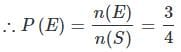Test: Probability Theory - 1 - Question 2

### From a pack of 52 cards, two cards are drawn together at random. What is the probability of both the cards being kings?

Detailed Solution for Test: Probability Theory - 1 - Question 2

Let S be the sample space
Then,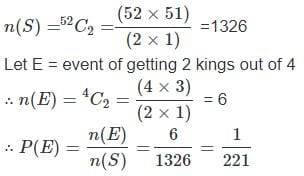Test: Probability Theory - 1 - Question 3

### A bag contains 6 black and 8 white balls. One ball is drawn at random. What is the probability that the ball drawn is white?

Detailed Solution for Test: Probability Theory - 1 - Question 3

Let number of balls = (6+8)=14
Number of white balls = 8P (drawing a white ball)
= 8/14 = 4/7

Test: Probability Theory - 1 - Question 4

Two cards are drawn together from a pack of 52 cards. The probability that one is a spade and one is a heart, is:

Detailed Solution for Test: Probability Theory - 1 - Question 4

Let S be the sample space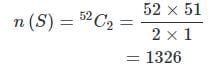Let E = event of getting 1 spade and 1 heart
∴ n(E) = number of ways of choosing 1 spade out of 13 and 1 heart out of 13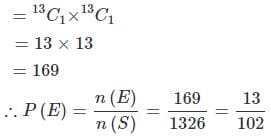Test: Probability Theory - 1 - Question 5

A card is drawn from a pack of 52 cards. The probability of getting a queen of club or a king of heart is:

Detailed Solution for Test: Probability Theory - 1 - Question 5

Here, n(S) = 52
Let E = event of getting a queen of club or a king of heart
Then, n(E) = 2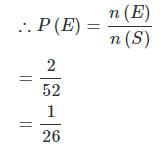Test: Probability Theory - 1 - Question 6

A bag contains 2 red, 3 green and 2 blue balls. Two balls are drawn at random. What is the probability that none of the balls drawn is blue?

Detailed Solution for Test: Probability Theory - 1 - Question 6

Total number of balls
= (2 + 3 + 2)
= 7
Let S be the sample space
Then, n(S) = Number of ways of drawing 2 balls out of 7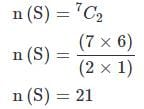Let E = Event of 2 balls, none of which is blue
∴ n(E) = Number of ways of drawing 2 balls out of (2 + 3) balls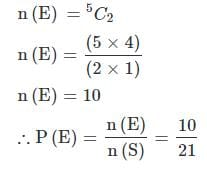Test: Probability Theory - 1 - Question 7

What is the probability of getting a sum 9 from two throws of a dice?

Detailed Solution for Test: Probability Theory - 1 - Question 7

In two throws of a dice, n(S) = (6 x 6) = 36
Let E = event of getting a sum
= {(3, 6), (4, 5), (5, 4), (6, 3)}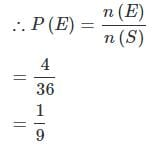Test: Probability Theory - 1 - Question 8

Two dice are thrown simultaneously. What is the probability of getting two numbers whose product is even?

Detailed Solution for Test: Probability Theory - 1 - Question 8

In a simultaneous throw of two dice, we have n(S) = (6 x 6) = 36

Then, E = {(1, 2), (1, 4), (1, 6), (2, 1), (2, 2), (2, 3), (2, 4), (2, 5), (2, 6), (3, 2), (3, 4), (3, 6), (4, 1), (4, 2), (4, 3), (4, 4), (4, 5), (4, 6), (5, 2), (5, 4), (5, 6), (6, 1), (6, 2), (6, 3), (6, 4), (6, 5), (6, 6)}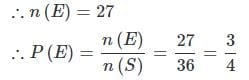Test: Probability Theory - 1 - Question 9

In a lottery, there are 10 prizes and 25 blanks. A lottery is drawn at random. What is the probability of getting a prize?

Detailed Solution for Test: Probability Theory - 1 - Question 9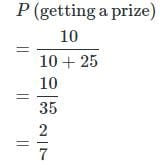Test: Probability Theory - 1 - Question 10

Two dice are tossed. The probability that the total score is a prime number is:

Detailed Solution for Test: Probability Theory - 1 - Question 10

Clearly, n(S) = (6 x 6) = 36
Let E = Event that the sum is a prime number.Then
E = {(1, 1), (1, 2), (1, 4), (1, 6), (2, 1), (2, 3), (2, 5), (3, 2), (3, 4), (4, 1), (4, 3), (5, 2), (5, 6), (6, 1), (6, 5)}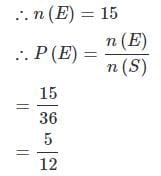## Statistics for SSC CGL

69 videos|90 docs|16 tests
Information about Test: Probability Theory - 1 Page
In this test you can find the Exam questions for Test: Probability Theory - 1 solved & explained in the simplest way possible. Besides giving Questions and answers for Test: Probability Theory - 1, EduRev gives you an ample number of Online tests for practice

## Statistics for SSC CGL

69 videos|90 docs|16 tests